# Definite Integral : Area Between Curves

Integration နှင့် ပတ်သက်ပြီး အောက်ပါ သင်ခန်းစာ များကို ရေးသားခဲ့ပါသည်။ ယခုသင်ခန်းစာကို ဖော်ပြပါသင်ခန်းစာများနှင့် တွဲဖက်လေ့လာရမည် ဖြစ်ပါသည်။

#### Problems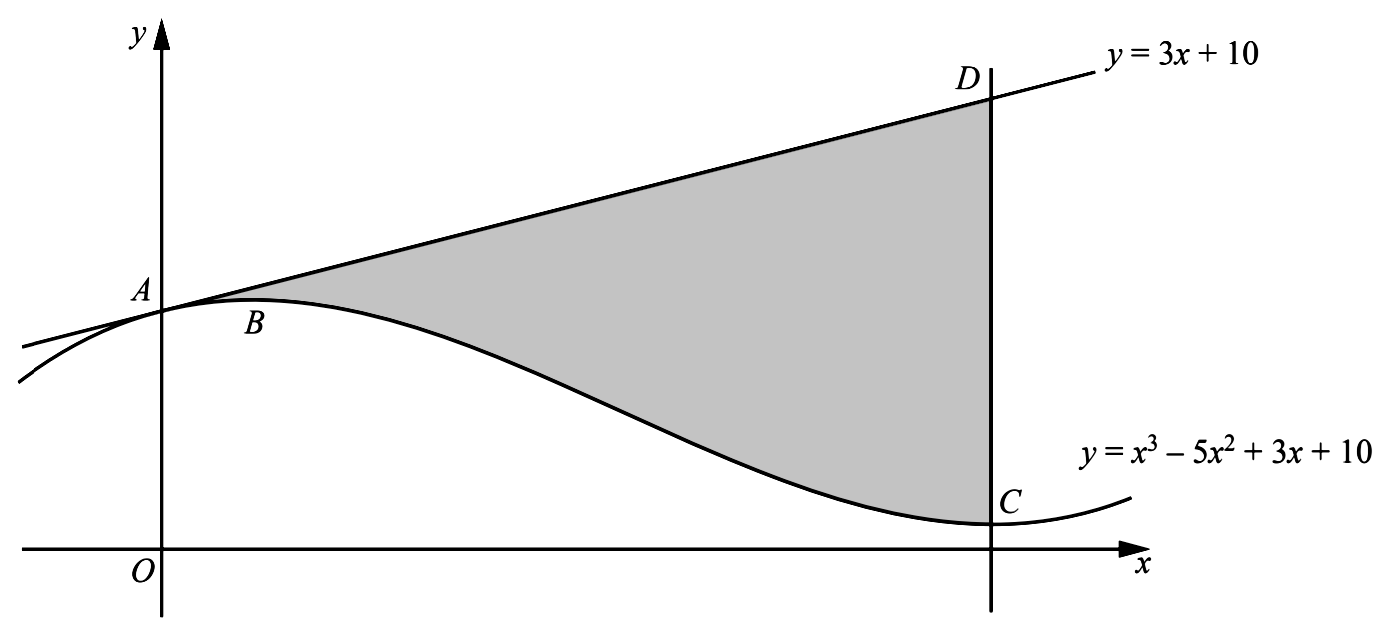1. The diagram shows parts of the line $y=3 x+10$ and the curve $y=x^{3}-5 x^{2}+3 x+10$. The line and the curve both pass through the point $A$ on the $y$ -axis. The curve has a maximum at the point $B$ and a minimum at the point $C$. The line through $C$, parallel to the $y$ -axis, intersects the line $y=3 x+10$ at the point $D$.
• Show that the line $A D$ is a tangent to the curve at $A$.
• Find the $x$ -coordinate of $B$ and of $C$.
• Find the area of the shaded region $A B C D$, showing all your working.

2. Line: $y=3 x+10$
When the line cuts $y$ -axis, $x=0$
$\therefore y=10$
Hence, the coordinate of the point $A$ is $(0,10)$
Curve: $y=x^{3}-5 x^{2}+3 x+10$
$y^{\prime}=3 x^{2}-10 x+3$
At $x=0, y^{\prime}=3$
The equation of tangent to the curve at $(0,10)$ is
$y-10=3 x$
$y=3 x+10$
At turning points, $y^{\prime}=0$
$\therefore \quad 3 x^{2}-10 x+3=0$
$(3 x-1)(x-3)=0$
When $x=\dfrac{1}{3}, y=\dfrac{283}{27}$
When $x=3, y=1$
$y^{\prime \prime}=9 x-10$
When $x=\dfrac{1}{3}, y^{\prime \prime}=-7<0$
When $x=3, y^{\prime \prime}=17>0$
$\therefore \quad B=\left(\dfrac{1}{3}, \dfrac{283}{27}\right)$ and $C=(3,1)$
$\quad\quad$ Area of shaded region
$=\displaystyle\int_{0}^{3}\left[3 x+10-\left(x^{3}-5 x^{2}+3 x+10\right)\right]\ d x$
$=\displaystyle\int_{0}^{3}\left(-x^{3}+5 x^{2}\right)\ d x$
$=\left[-\dfrac{x^{4}}{4}+\dfrac{5 x^{3}}{3}\right]_{0}^{3}$
$=45-\dfrac{8-1}{4}$
$=\dfrac{180-81}{4}$
$=\dfrac{99}{4}$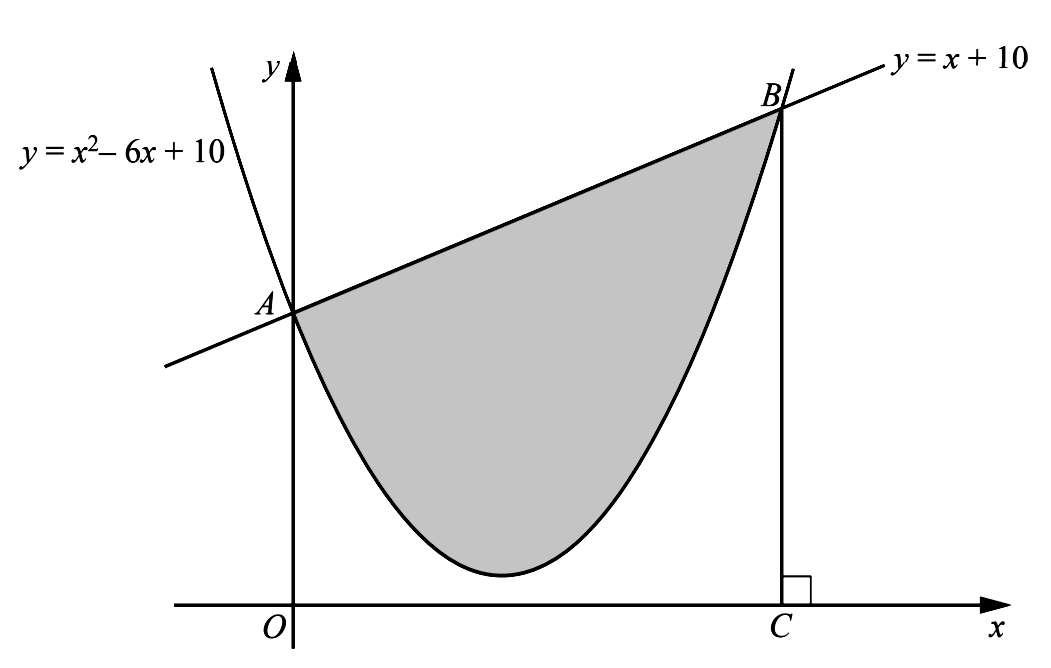3. The graph of $y=x^{2}-6 x+10$ cuts the $y$ -axis at $A$. The graphs of $y=x^{2}-6 x+10$ and $y=x+10$ cut one another at $A$ and $B$. The line $B C$ is perpendicular to the $x$ -axis. Calculate the area of the shaded region enclosed by the curve and the line $A B$, showing all your working.

4. Line: $y_{1}=x+10$
Curve: $y=x^{2}-6 x+10$
When the line intersect the curves,
$y_{1}=y_{2}$
$\therefore\ x^{2}-6 x+10=x+10$
$x^{2}-7 x=0$
$x(x-7)=0$
$x=0$ or $x=7$
When $x=0, y=10$
When $x=7, y=17$
$\therefore A=(0,10)$ and $B=(7,17) .$
$\quad$ Area of shaded region
$=\displaystyle\int_{0}^{7}\left(y_{1}-y_{2}\right)\ d x$
$=\displaystyle\int_{0}^{7}\left(7 x-x^{2}\right)\ d x$
$=\left[\dfrac{7 x^{2}}{2}-\dfrac{x^{3}}{3}\right]_{0}^{7}$
$=\dfrac{7^{3}(3-2)}{6}=\dfrac{343}{6}$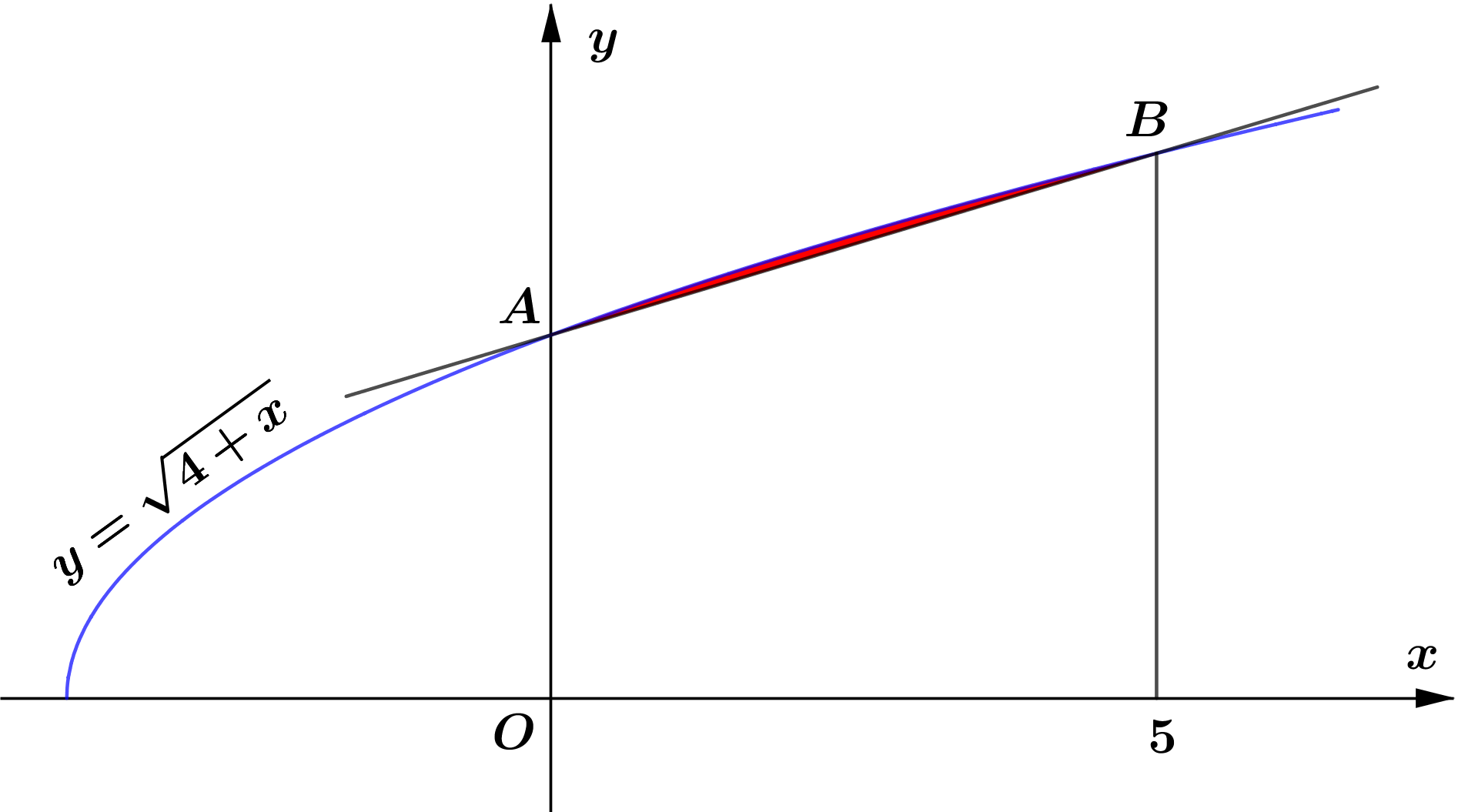5. The diagram shows the graph of $y=\sqrt{4+x}$, which meets the $y$ -axis at the point $A$ and the line $x=5$ at the point $B$. Find the area of the region enclosed by the curve and the straight line $A B$.

6. Curve: $y_{1}=\sqrt{4+x}$
When $x=0, \quad y=\sqrt{4}=2$
When $x=5, \quad y=\sqrt{9}=3$
$\therefore A=(0,2)$ and $B=(5,3)$
$\therefore$ Equation of $A B$ is
$y_{2}=\dfrac{3-2}{5-0} x+2$
$\quad =\dfrac{x}{5}+2$
\begin{aligned} & \text { Area of required region } \\\\ =& \displaystyle\int_{0}^{5}\left(y_{1}-y_{2}\right)\ d x \\\\ =& \displaystyle\int_{0}^{5}\left(\sqrt{4+x}-\dfrac{x}{5}-2\right)\ d x \\\\ =& \displaystyle\int_{0}^{5}(4+x)^{1/2}\ d x-\displaystyle\int_{0}^{5} \dfrac{x}{5} d x-\displaystyle\int_{0}^{5} 2\ d x\\\\ =& \displaystyle\int_{0}^{5}(4+x)^{1 / 2}\ d(4+x)-\displaystyle\int_{0}^{5} \dfrac{x}{5}\ d x-\displaystyle\int_{0}^{5} 2\ d x[\because d(4+x)=d x] \\\\ =& \left[\dfrac{2}{3}(4+x)^{3 / 2}\right]_{0}^{5}-\left[\dfrac{x^{2}}{10}\right]_{0}^{5}-[2 x]_{0}^{5} \\\\ =& \dfrac{2}{3}\left[9^{3 / 2}-4^{3 / 2}\right]-\dfrac{25}{10}-10 \\\\ =& \dfrac{2}{3}[27-8]-\dfrac{5}{2}-10 \\\\ =& \dfrac{38}{3}-\dfrac{25}{2}=\dfrac{1}{6} \end{aligned}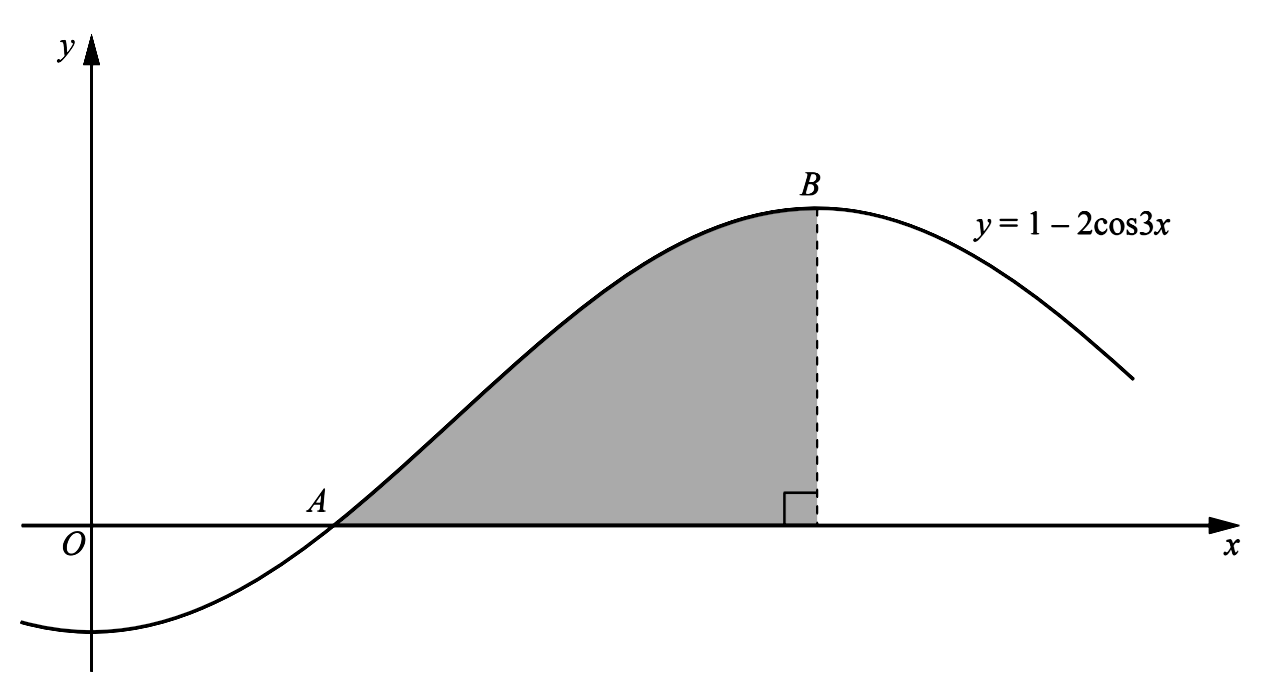7. The diagram shows part of the graph of $y=1-2 \cos 3 x$, which crosses the $x$ -axis at the point $A$ and has a maximum at the point $B$.
• Find the coordinates of $A$.
• Find the coordinates of $B$.
• Showing all your working, find the area of the shaded region bounded by the curve, the $x$ -axis and the perpendicular from $B$ to the $x$ -axis.

8. $y=1-2 \cos 3 x$
When $y=0,$
$1-2 \cos 3 x=0$
$\cos 3 x=\dfrac{1}{2}$
By the diagram,
$3 x=\dfrac{\pi}{3}$
$x=\dfrac{\pi}{9}$
$\therefore \quad A=\left(\dfrac{\pi}{9}, 0\right)$
Since $-1 \le \cos 3 x \le 1$
The maximum value of $y=3$, when
$\cos 3 x=-1$
By the diagran,
$3 x=\pi$
$x=\dfrac{\pi}{3}$
$\therefore B=\left(\dfrac{\pi}{3}, 3\right)$
$\quad$ Area of shaded region
\begin{aligned} =& \displaystyle\int_{\frac{\pi}{9}}^{\frac{\pi}{3}}(1-2 \cos 3 x) \ d x \\\\ =& \displaystyle\int_{\frac{\pi}{9}}^{\frac{\pi}{3}} 1 \ d x-\dfrac{2}{3} \displaystyle\int_{\frac{\pi}{9}}^{\frac{\pi}{3}} \cos 3 x\ d(3 x) \\\\ =&[x]_{\frac{\pi}{9}}^{\frac{\pi}{3}}-\left[\dfrac{2}{3} \sin 3 x\right]_{\frac{\pi}{9}}^{\frac{\pi}{3}}\\\\ =&\left(\dfrac{\pi}{3}-\dfrac{\pi}{9}\right)-\dfrac{2}{3}\left[\sin \pi-\sin \dfrac{\pi}{3}\right] \\\\ =&\dfrac{2 \pi}{9}-\dfrac{2}{3}\left[0-\dfrac{\sqrt{3}}{2}\right] \\\\ =&\dfrac{2 \pi}{9}+\dfrac{\sqrt{3}}{3} \end{aligned}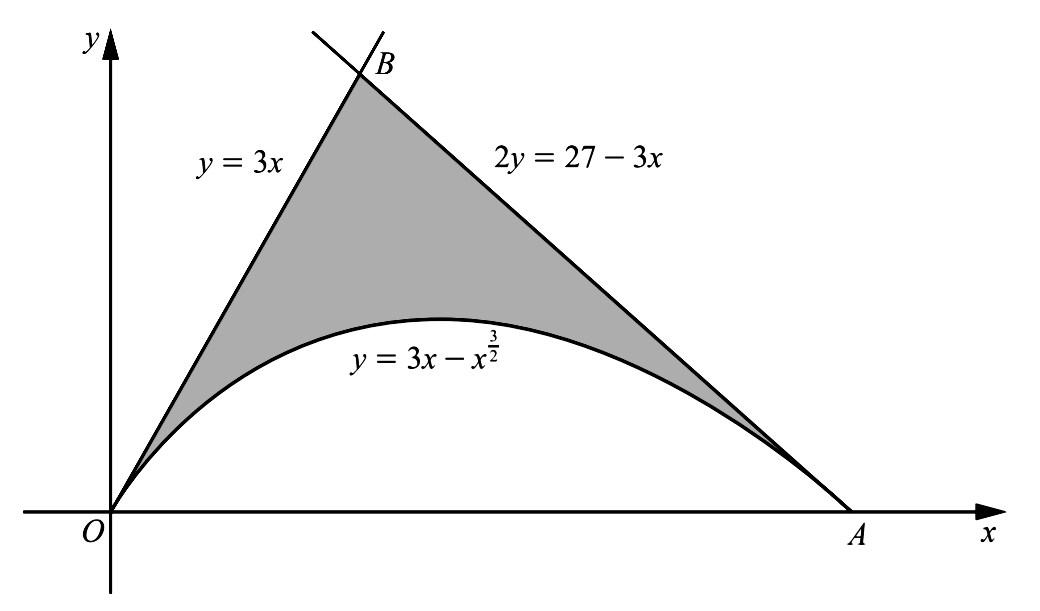9. The diagram shows part of the curve $y=3 x-x^{\frac{3}{2}}$ and the lines $y=3 x$ and $2 y=27-3 x$. The curve and the line $y=3 x$ meet the $x$ -axis at $O$ and the curve and the line $2 y=27-3 x$ meet the $x$ -axis at $A$.
• Find the coordinates of $A$.
• Verify that the coordinates of $B$ are $(3,9)$
• Find the area of the shaded region.

10. Line1: $y_{1}=3 x$
Line2: $2 y_{2}=27-3 x$
When line 2 cuts $x$ -axis,
$\quad\quad y_{2}=0$
$\therefore\ 27-3 x=0$
$\hspace{1.7cm} x=3$
$\therefore\ A=(9,0)$
At the point of intersection of Line 1 and line 2,
$\quad\quad y_{1}=y_{2}$
$\therefore\ 2(3 x)=27-3 x$
$\quad\quad 9 x=27$
$\quad\quad x=3$
$\therefore\ y=3(3)=9$
$\therefore\ B=(3,9)$
$\quad\quad$ Area of shaded region
\begin{aligned} &= \text { area of } \triangle O A B - \text { area under curve } \\\\ &= \dfrac{1}{2}(9)(9)-\displaystyle\int_{0}^{9}\left(3 x-x^{\frac{3}{2}}\right)\ d x \\\\ &= \dfrac{81}{2}-\left[\dfrac{3 x^{2}}{2}-\dfrac{2 x^{5 / 2}}{5}\right]_{0}^{9} \\\\ &= \dfrac{81}{2}-\dfrac{3(81)}{2}+\frac{2(243)}{5} \\\\ &= \dfrac{81}{5} \end{aligned}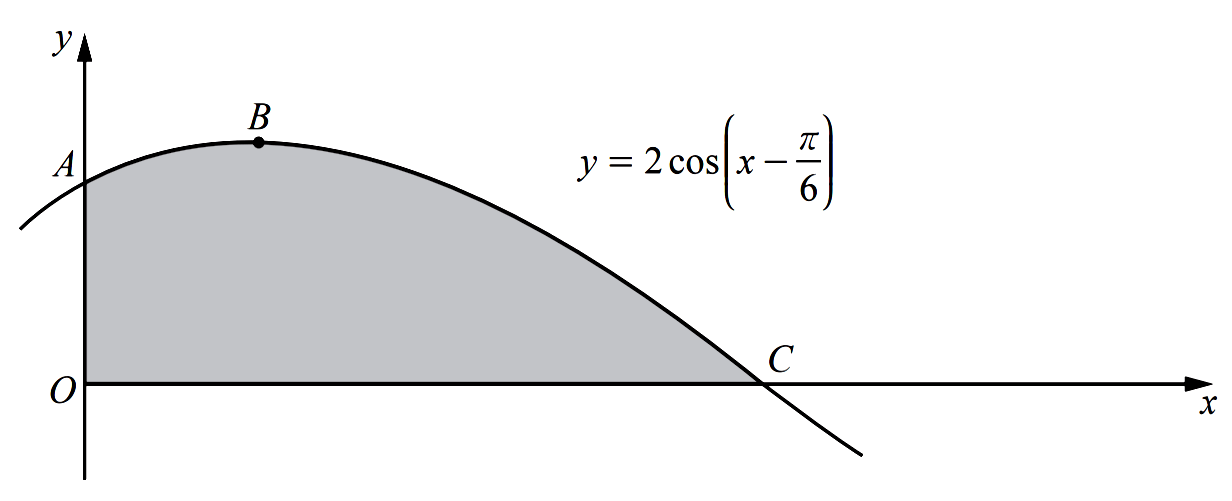11. The diagram shows part of the graph of $y=2 \cos \left(x-\dfrac{\pi}{6}\right)$. The graph intersects the $y$ -axis at the point $A$, has a maximum point at $B$ and intersects the $x$ -axis at the point $C$.
• Find the coordinates of $A$.
• Find the coordinates of $B$.
• Find the coordinates of $C$.
• Find $\displaystyle\int 2 \cos \left(x-\dfrac{\pi}{6}\right) \mathrm{d} x$.
• Hence find the area of the shaded region.

12. $y=2 \cos \left(x-\dfrac{\pi}{6}\right)$
When $x=0$,
$y=2 \cos \left(-\dfrac{\pi}{6}\right)$
$=\sqrt{3}$
$\therefore\ A=(0, \sqrt{3})$
The maximum value of $y=2 \cos \left(x-\dfrac{\pi}{6}\right)$ is $2$ when $\cos \left(x-\dfrac{\pi}{6}\right)=1$
$x-\dfrac{\pi}{6}=0$
$x=\dfrac{\pi}{6}$
$B=\left(\dfrac{\pi}{6}, 0\right)$
When $y=0,$
$2 \cos \left(x-\dfrac{\pi}{6}\right)=0$
$\cos \left(x-\dfrac{\pi}{6}\right)=0$
$x-\dfrac{\pi}{6}=\dfrac{\pi}{2}$
$x=\dfrac{\pi}{2}+\dfrac{\pi}{6}$
$\therefore x=\dfrac{2 \pi}{3}$
$\therefore C=\left(\dfrac{2 \pi}{3}, 0\right)$
$\displaystyle\int 2 \cos \left(x-\dfrac{\pi}{6}\right)\ d x$
let $u=x-\dfrac{\pi}{6}$
$\therefore\ d u=d x$
\begin{aligned} \displaystyle\int 2 \cos \left(x-\dfrac{\pi}{6}\right)\ d x =&\displaystyle\int 2 \cos u\ d u \\\\ =&2 \sin u+C, c=\text { constant } \\\\ =&2 \sin \left(x-\dfrac{\pi}{6}\right)+C \end{aligned}
\begin{aligned} & \text { Area of shaded region } \\\\ =& \displaystyle\int_{0}^{\dfrac{2 \pi}{3}} 2 \cos \left(x-\dfrac{\pi}{6}\right)\ d x \\\\ =&\left[2 \sin \left(x-\dfrac{\pi}{6}\right)\right]_{0}^{\frac{2 \pi}{3}} \\\\ =& 2\left[\sin \dfrac{\pi}{2}-\sin \left(-\dfrac{\pi}{6}\right)\right]\\\\ =& 2\left[\sin \dfrac{\pi}{2}+\sin \dfrac{\pi}{6}\right] \\\\ =& 2\left[1+\dfrac{1}{2}\right]= 3 \\\\ \end{aligned}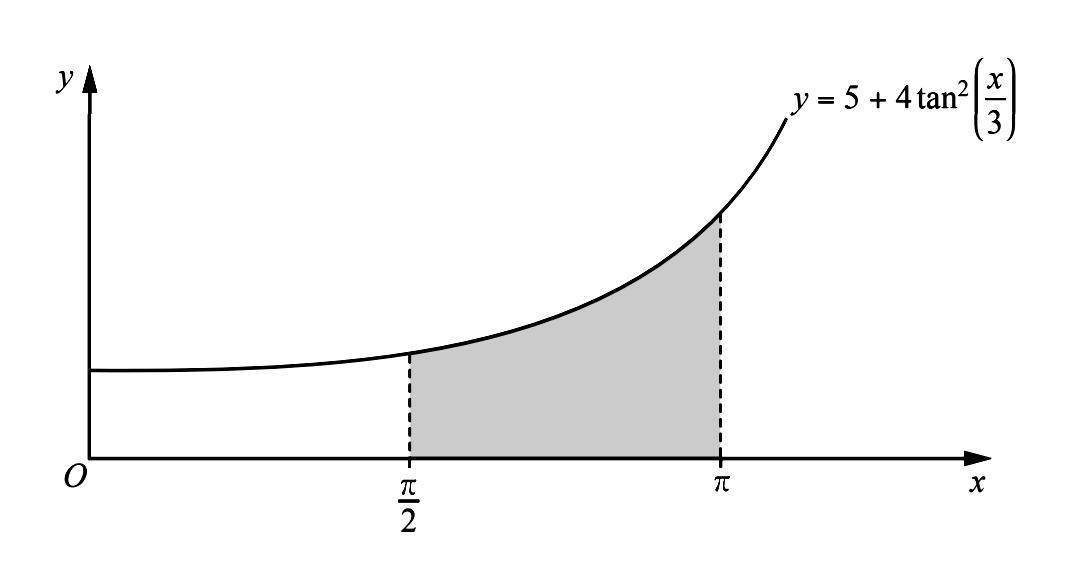13. The diagram shows part of the curve $y=5+4 \tan ^{2}\left(\dfrac{x}{3}\right)$. Hence, find the exact area of the shaded region enclosed by the curve, the $x$ -axis and the lines $x=\dfrac{\pi}{2}$ and $x=\pi$

14. \begin{aligned} y&=5+4 \tan ^{2}\left(\dfrac{x}{3}\right) \\\\ &=5+4\left(\sec ^{2}\left(\dfrac{x}{3}\right)-1\right) \\\\ &=4 \sec ^{2}\left(\dfrac{x}{3}\right)+1 \end{aligned}
\begin{aligned} &\text { Area of shaded region } \\\\ = & \displaystyle\int_{\dfrac{\pi}{2}}^{\pi}\left[5+4 \tan ^{2}\left(\dfrac{x}{3}\right)\right]\ d x \\\\ = & \displaystyle\int_{\dfrac{\pi}{2}}^{\pi}\left(4 \sec ^{2}\left(\dfrac{x}{3}\right)+1\right)\ d x\\\\ = & 4 \displaystyle\int_{\dfrac{\pi}{2}}^{\pi} \sec ^{2}\left(\dfrac{x}{3}\right)\ d x+\displaystyle\int_{\frac{\pi}{2}}^{\pi}1\ d x \\\\ = & \left[\dfrac{4}{3} \tan \left(\dfrac{x}{3}\right)\right]_{\frac{\pi}{2}}^{\pi}+\left[x\right]_{\frac{\pi}{2}}^{\pi} \\\\ = & \frac{4}{3}\left[\tan \dfrac{\pi}{3}-\tan \dfrac{\pi}{6}\right]+\left[\pi-\dfrac{\pi}{2}\right] \\\\ = & 4\left[\sqrt{3}-\dfrac{\sqrt{3}}{3}\right]+\dfrac{\pi}{2} \\\\ = & 8 \sqrt{3}+\dfrac{\pi}{2} \end{aligned}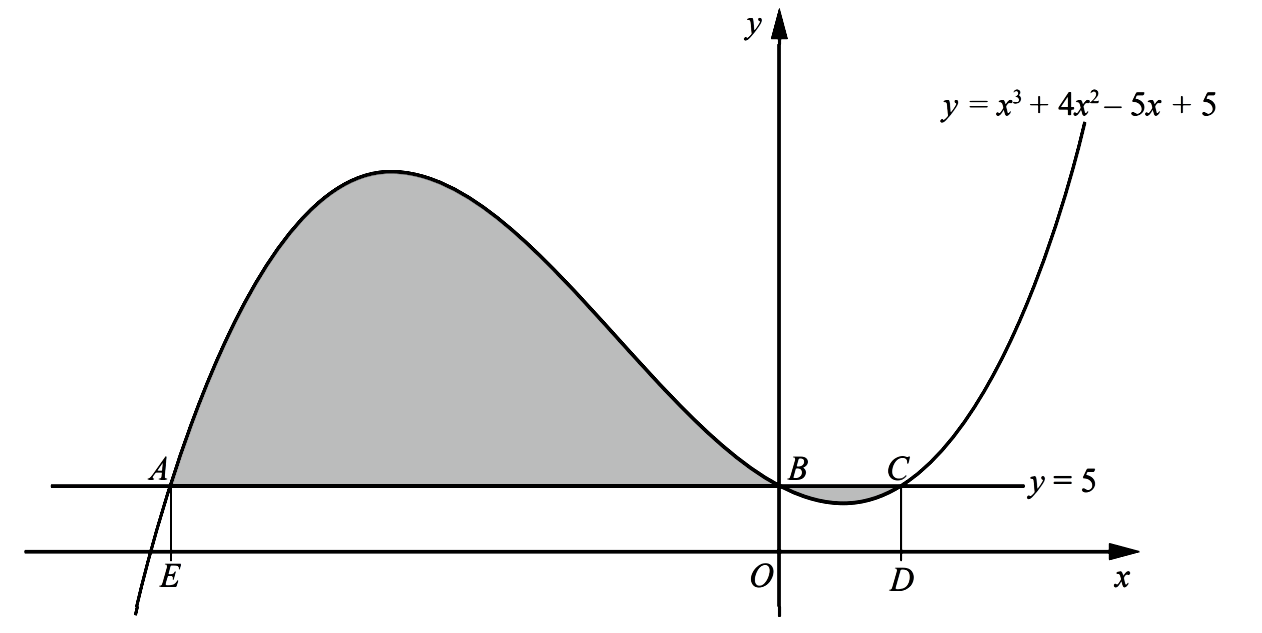15. The diagram shows part of the curve $y=x^{3}+4 x^{2}-5 x+5$ and the line $y=5$. The curve and the line intersect at the points $A, B$ and $C .$ The points $D$ and $E$ are on the $x$ -axis and the lines $A E$ and $C D$ are parallel to the $y$ -axis.Calculate the total area of the shaded regions enclosed between the line and the curve. You must show all your working.

16. $y=x^{3}+4 x^{2}-5 x+5, y_{2}=5$
When $y=y_{2}$
$x^{3}+4 x^{2}-5 x+5=5$
$x^{3}+4 x^{2}-5 x=0$
$x\left(x^{2}+4 x-5\right)=0$
$\therefore x(x+5)(x-1)=0$
$\therefore x=-5$ or $x=0$ or $x=7$
$\quad\quad$ area of shaded region
\begin{aligned} &=\displaystyle\int_{-5}^{0}\left(y_{1}-y_{2}\right)\ d x+\displaystyle\int_{0}^{1}\left(y_{2}-y_{1}\right)\ d x\\\\ &=\displaystyle\int_{-5}^{0}\left(x^{3}+4 x^{2}-5 x\right)\ d x+\int_{0}^{1}\left(-x^{3}-4 x^{2}+5 x\right)\ d x \\\\\\ &=\left[\dfrac{1}{4} x^{4}+\dfrac{4}{3} x^{3}-\dfrac{5}{2} x^{2}\right]_{-5}^{0}+\left[-\dfrac{1}{4} x^{3}-\dfrac{4}{3} x+\dfrac{5}{2} x^{2}\right]_{0}^{1} \\\\ &=-\left[\dfrac{1}{4}(625)+\dfrac{4}{3}(-125)-\dfrac{5}{2}(25)\right]+\left[-\dfrac{1}{4}-\dfrac{4}{3}+\dfrac{5}{2}\right] \\\\ &=\dfrac{443}{6} \end{aligned}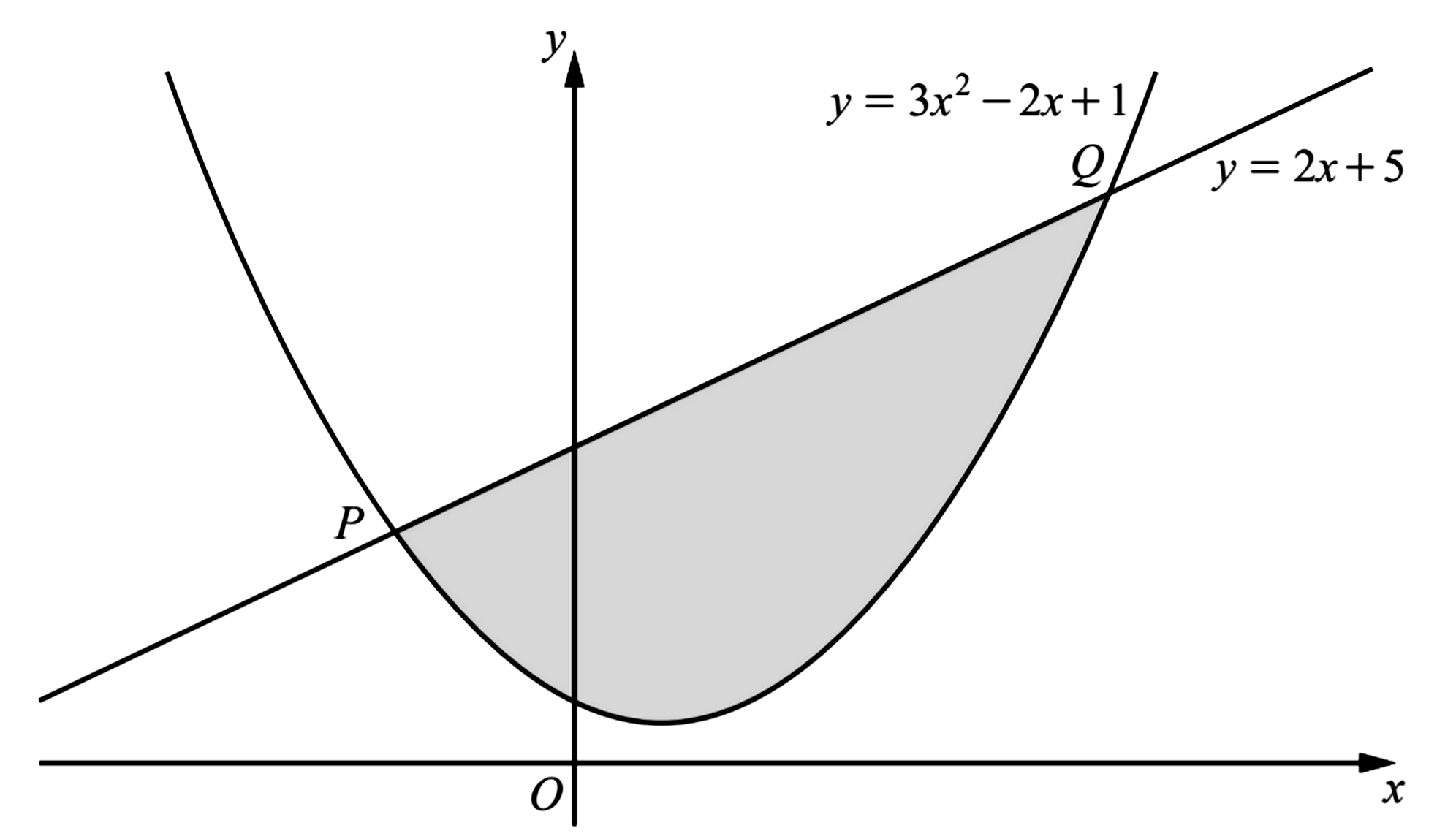17. The diagram shows the curve $y=3 x^{2}-2 x+1$ and the straight line $y=2 x+5$ intersecting at the points $P$ and $Q$. Showing all your working, find the area of the shaded region.

18. $y_{1}=2 x+5$
$y_{2}=3 x^{2}-2 x+1$
When $y_{1}=y_{2}$,
$2 x+5=3 x^{2}-2 x+1$
$\therefore\ 3 x^{2}-4 x-4=0$
$(3 x+2)(x-2)=0$
$x=-\dfrac{2}{3}$ or $x=2$
When $x=-\dfrac{2}{3}, y=-\dfrac{4}{3}+5=\dfrac{11}{3}$
When $x=2, y=4+5=9$
$\therefore P=\left(-\dfrac{2}{3}, \dfrac{11}{3}\right)$ and $Q=(2,9)$
$\quad\quad$ Area of shaded region
$=\displaystyle\int_{-2 / 3}^{2}\left(y_{1}-y_{2}\right)\ d x$
$=\displaystyle\int_{-2 / 3}^{2}\left(-3 x^{2}+4 x+4\right)\ d x$
$=\left[-x^{3}+2 x^{2}+4 x\right]_{-2 / 3}^{2}$
$=-8+8+8-\left(\dfrac{8}{27}+\dfrac{8}{9}-\dfrac{8}{3}\right)$
$=\dfrac{256}{27}$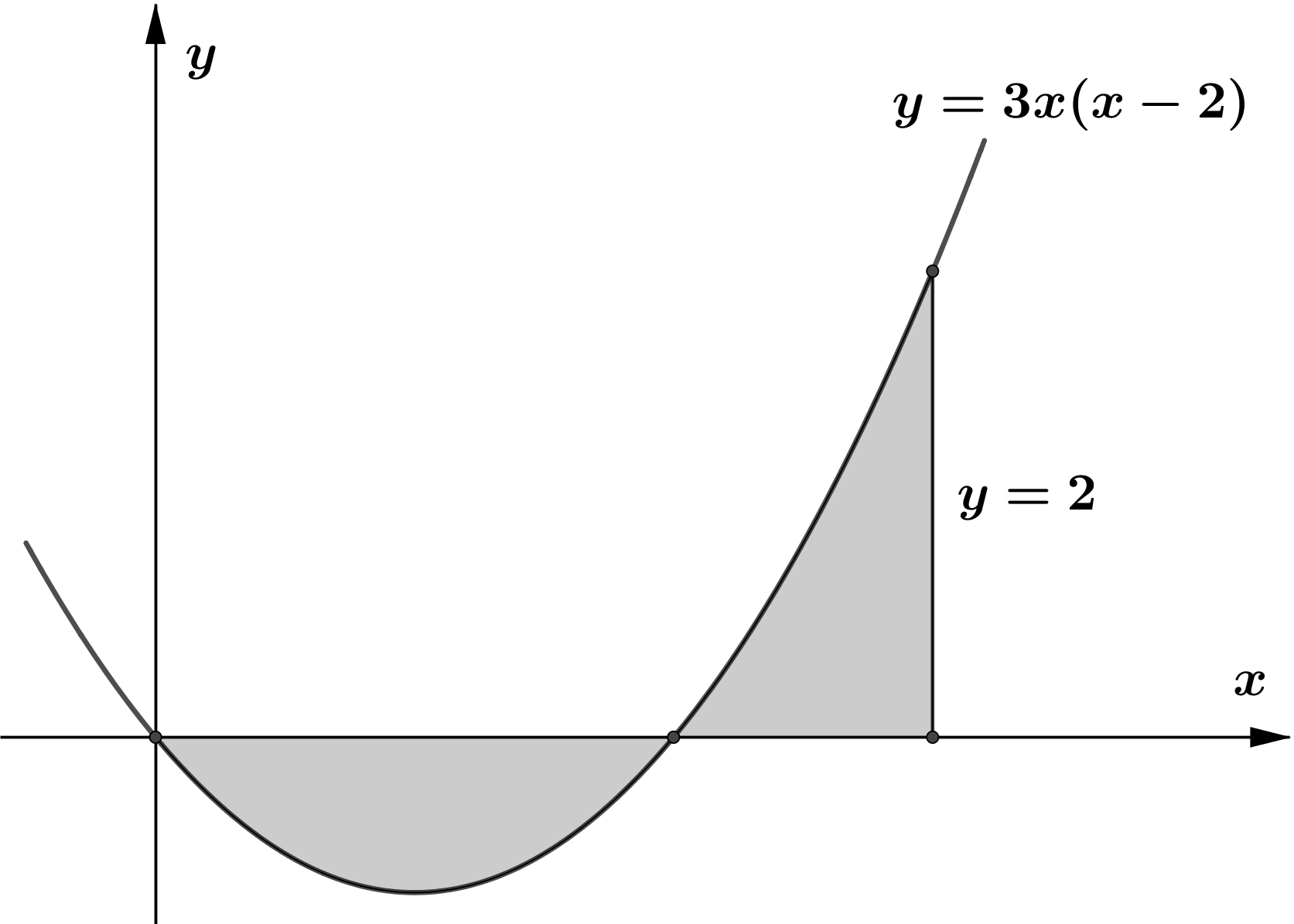19. The figure shows part of the curve $y=3 x(x-2) .$ Find the total area enclosed by the curve $y=3 x(x-2)$, the $x$ -axis and the line $x=3$

20. $y=3 x(x-2)=3 x^{2}-6 x$
When $y=0$,
$3 x(x-2)=0$
$\therefore x=0$ or $x=2$
The curve $y=3 x(x-2)$ cuts $x$ -axis at $(0,0)$ and $(2,0)$
$\quad\quad$ Area of shaded region
\begin{aligned} =& \displaystyle\int_{0}^{2}-y d x+\int_{2}^{3} y d x \\\\ =& \displaystyle\int_{0}^{2}\left(-3 x^{2}+6 x\right) d x+\displaystyle\int_{2}^{3}\left(3 x^{2}-6 x\right) d x \\\\ =&\left[-x^{3}+3 x^{2}\right]_{0}^{2}+\left[x^{3}-3 x^{2}\right]_{2}^{3} \\\\ =&[-8+12]+[27-27-8+12] \\\\ =& 8 \end{aligned}21. The diagram shows the curve $y=4+3 x-x^{2}$ intersecting the positive $x$ -axis at the point $A$. The line $y=m x+8$ is a tangent to the curve at the point $B$. Find
• the coordinates of $A$,
• the value of $m$
• the coordinates of $B$,

22. Curve: $y_{1}=4+3 x-x^{2}$
Line: $y_{2}=m x+8$
When the curve ents $x$ -axis, $y=0$
$\therefore\ 4+3 x-x^{2}=0$
$(1+x)(4-x)=0$
$\therefore\ x=-1$ or $x=4$
$\therefore\ A=(4,0)$
Gradient of tangent to the curve $=y_{1}^{\prime}=3-2 x$
$\therefore m=3-2 x$
At the point of contact,
$y_{1}=y_{2}$
$\therefore 4+3 x-x^{2}=(3-2 x) x+8$
$x^{2}-4=0$
$x^{2}=4$
$x=\pm 2$
By the diagran,
$\quad\quad x=2$
$\therefore\ m=3-2(2)=-1$
$y_{2}=8-x$
When $x=2$
$y=-1(2)+8$
$\quad =6$
$\therefore\ B=(2,6)$
When the tangent cuts $x$ -axis,
$8-x=0 \Rightarrow x=8$.
$\quad\quad$ Area of shaded region
\begin{aligned} =& \displaystyle\int_{2}^{4}\left(y_{2}-y_{1}\right)\ d x+\int_{4}^{8} y_{2}\ d x \\\\ =& \displaystyle\int_{2}^{4}\left(x^{2}-4 x+4\right)\ d x+\int_{4}^{8}(8-x)\ d x \\\\ =&\left[\dfrac{1}{3} x^{3}-2 x^{2}+4 x\right]_{2}^{4}+\left[8 x-\dfrac{1}{2} x^{2}\right]_{4}^{8} \\\\ =&\left[\dfrac{64}{3}-32+16-\dfrac{8}{3}+8-8\right]+[64-32-32+8] \\\\ =& \dfrac{32}{3} \end{aligned}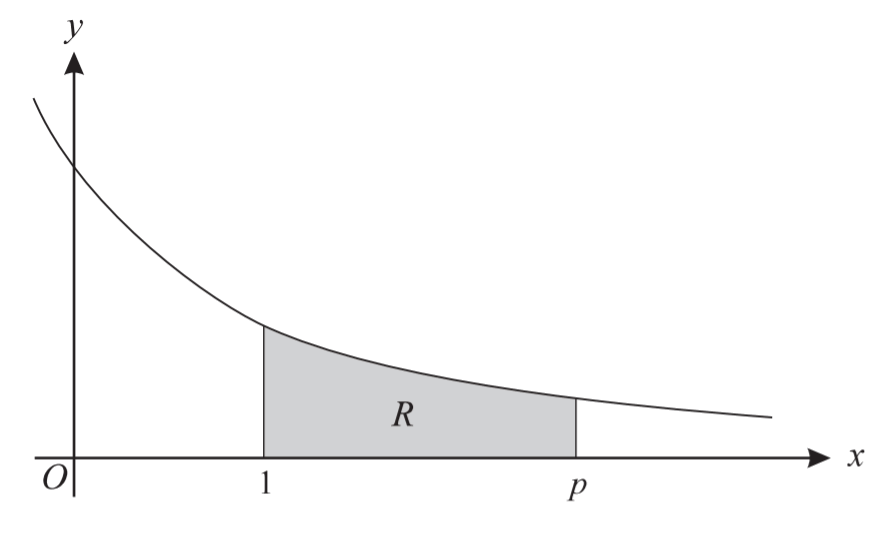23. The diagram shows part of the curve $y=\dfrac{1}{x+1}$. The shaded region $R$ is bounded by the curve and by the lines $x=1, y=0$ and $x=p$.
• Find, in terms of $p$, the area of $R$.
• Hence find, correct to 1 decimal place, the value of $p$ for which the area of $R$ is equal to 2 .

24. $\quad\quad$ Area of $R$
$= \displaystyle\int_{1}^{p} \dfrac{1}{x+1}\ d x$
$=[\ln |x+1|]_{1}^{p}$
$= \ln |p+1|-\ln 2$
By the problem,
$\ln |p+1|-\ln 2=2$
$\ln |p+1|=2+\ln 2$
$p+1=e^{2+\ln 2}$
$\therefore\ p =2 e^{2}-1=13.8$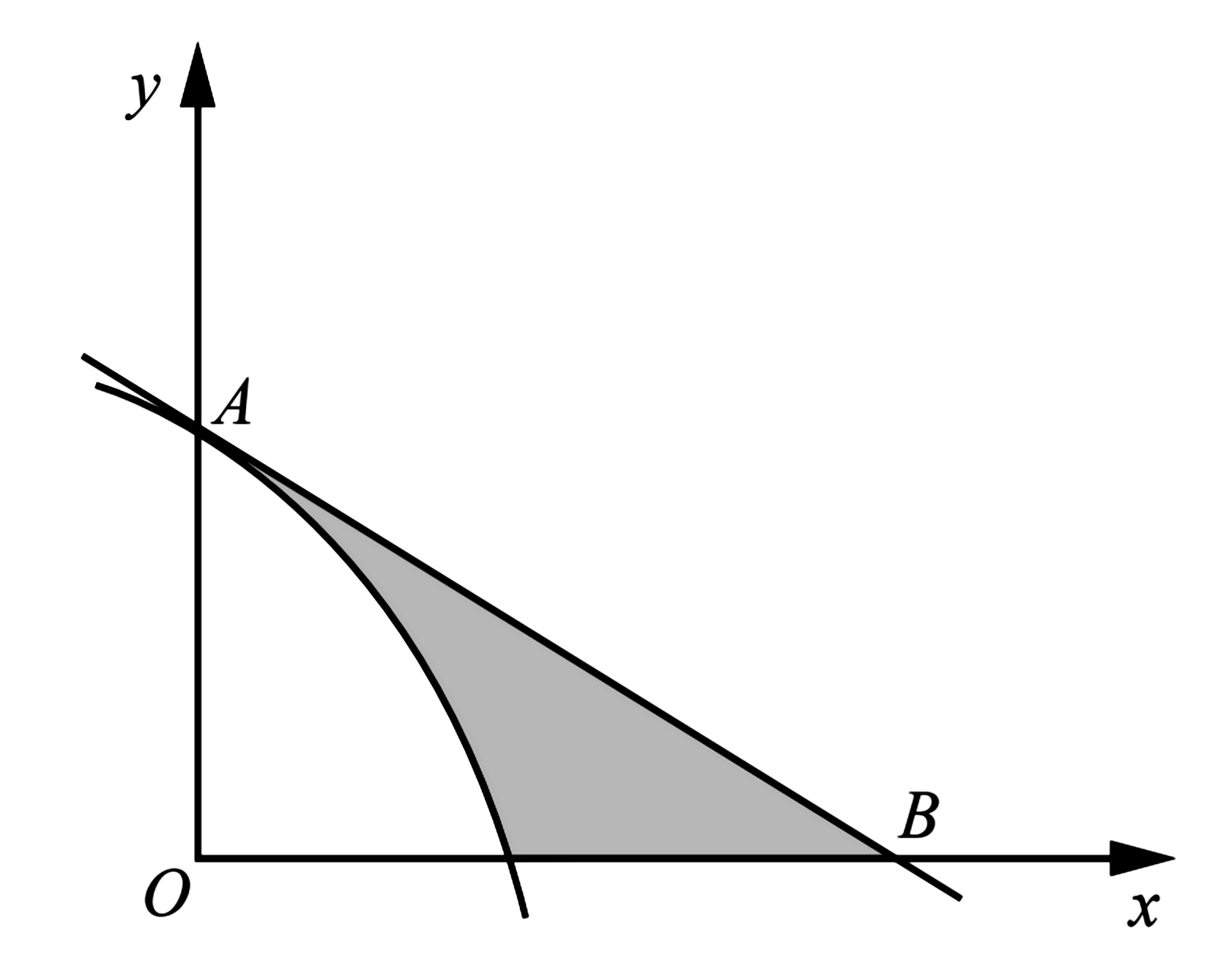25. The diagram, which is not drawn to scale, shows part of the graph of $y=8-\mathrm{e}^{2 x}$, crossing the $y$ -axis at $A$. The tangent to the curve at $A$ crosses the $x$ -axis at $B$. Find the area of the shaded region bounded by the curve, the tangent and the $x$ -axis.

26. Curve: $y=8-e^{2 x}$
When $x=0$
$y=8-e^{0}=7$
$\therefore\ A=(0,7)$
Gradient of tangent to the curve is
$y^{\prime}=-2 e^{2 x}$
When $x=0, y^{\prime}=-2$
$\therefore$ Equation of targent at $A$ is
$y=-2 x+7$
When the tangent cuts $x$ -axis,
$\quad y=0$
$-2 x+7=0$
$x=\dfrac{7}{2}$
$\therefore B=\left(\dfrac{7}{2}, 0\right)$
$8-e^{2 x}=0$
$e^{2 x}=8$
$2 x=\ln 8$
$x=\dfrac{\ln 8}{2}$
$\quad\quad$ Area of shaded region
$=\displaystyle\int_{0}^{\frac{\ln 8}{2}}\left(-2 x+7-8+e^{2 x}\right)\ d x+\displaystyle\int_{\frac{\ln 8}{2}}^{7 / 2}(-2 x+7)\ d x$
$=\left[-x^{2}-x+\dfrac{1}{2} e^{2 x}\right]_{0}^{\frac{\ln 8}{2}}+\left[-x^{2}+7 x\right]_{\frac{\ln 8}{2}}^{7 / 2}$
$=-\left(\dfrac{\ln 8}{2}\right)^{2}-\dfrac{\ln 8}{2}+4-\dfrac{1}{2}-\dfrac{49}{4}+\dfrac{49}{2}+\left(\dfrac{\ln 8}{2}\right)^{2}-\dfrac{7}{2} \ln 8$
$=7.4322$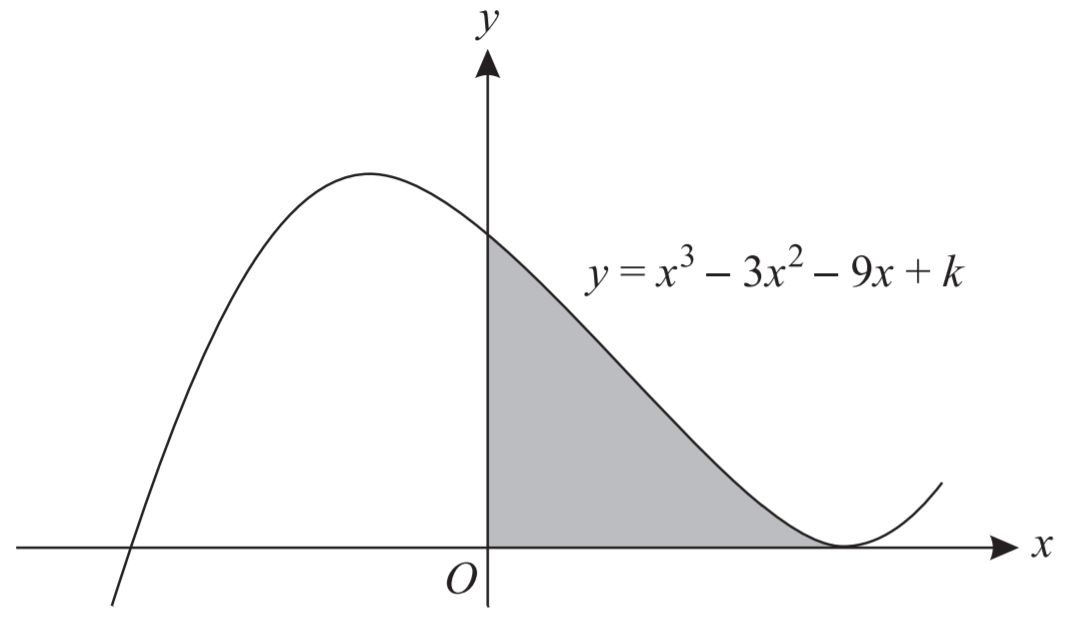27. The diagram shows the curve $y=x^{3}-3 x^{2}-9 x+k$, where $k$ is a constant. The curve has a minimum point on the $x$ -axis.
• Find the value of $k$.
• Find the area of the shaded region.

28. $y=x^{3}-3 x^{2}-9 x+k$
Let the mimiun porint be $(a, 0)$
$\therefore a^{3}-3 a^{2}-9 a+k=0$
$y^{\prime}=3 u^{2}-6 x-9$
At $(a, 0), y=0$
$\therefore 3 a^{2}-6 a-9=0$
$a^{2}-2 a-3=0$
$(a+1)(a-3)=0$
$\therefore a=-1$ or $a=3$
By the diagran, $a=3$
$\therefore$ minimun ponnt $=(3,0)$
When $a=3, k=27$.
$\therefore y=x^{3}-3 x^{2}-9 x+27 .$
$\quad\quad$Area of shaded region
$=\displaystyle\int_{0}^{3}\left(x^{3}-3 x^{2}-9 x+27\right)\ d x$
$=\left[\dfrac{x^{4}}{4}-x^{3}-\dfrac{9}{2} x^{2}+27 x\right]_{0}^{3}=\dfrac{135}{4}$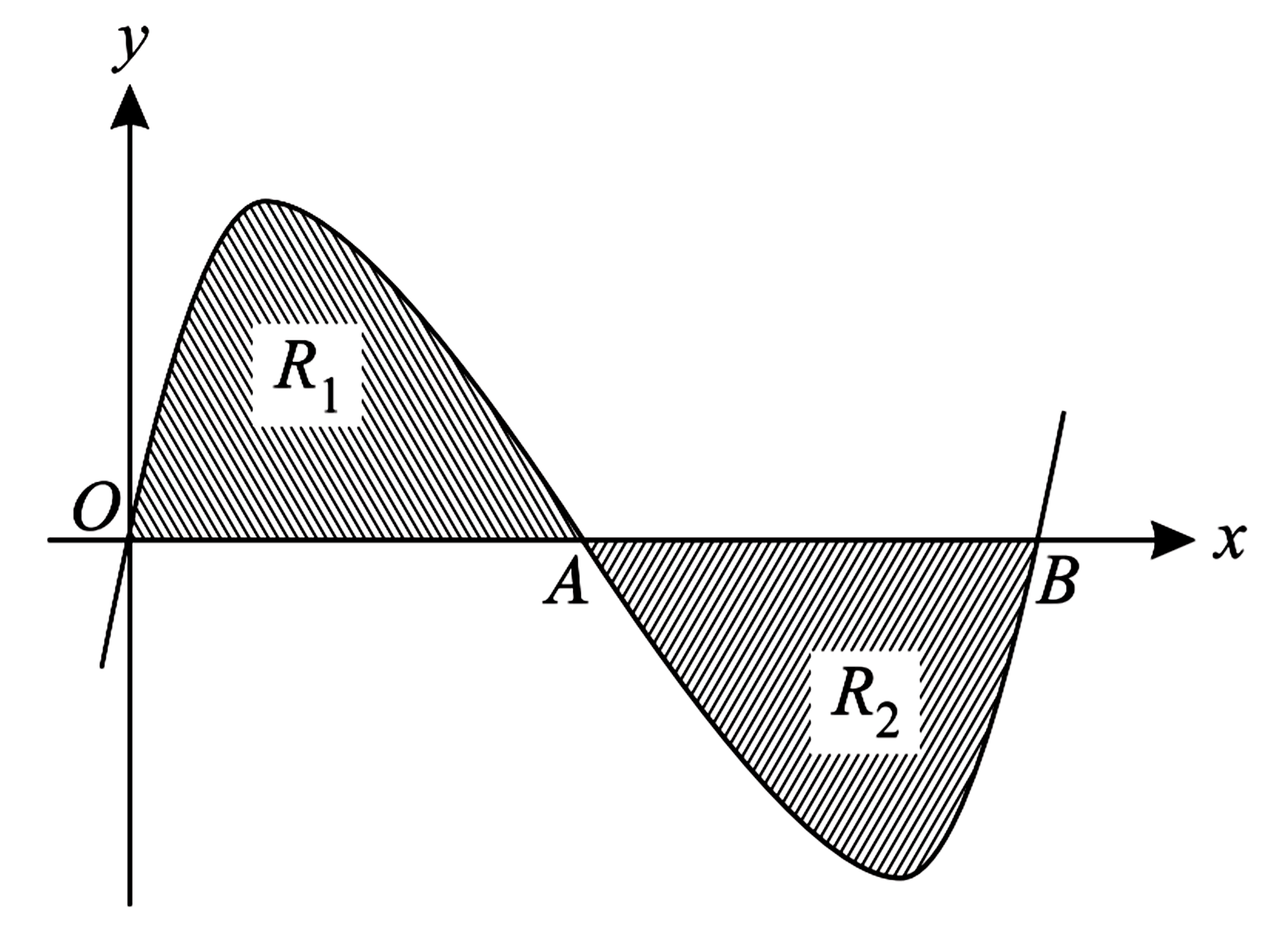29. The diagram shows the curve $y=x(x-1)(x-2)$, which crosses the $x$ -axis at the points $O(0,0)$, $A(1,0)$ and $B(2,0)$.
• The tangents to the curve at the points $A$ and $B$ meet at the point $C$. Find the $x$ -coordinate of $C$.
• Show by integration that the area of the shaded region $R_{1}$ is the same as the area of the shaded region $R_{2}$

30. Curve: $y=x(x-1)(x-2)$
$\hspace{1cm}y=x^{3}-3 x^{2}+2 x$
$\therefore y^{\prime}=3 x^{2}-6 x+2$
At $x=1, \quad y^{\prime}=-1$
At $x=2, \quad y^{\prime}=2$
Equation of tangent $A$ is
$y-0=-1(x-1)$
$y=1-x$
Equation of tangent at $B$ is
$y-0=2(x-2)$
$y=2 x-4$
Whent the two tangent intersect each other,
$1-x=2 x-4$
$x=\dfrac{5}{3}$
When $x=\dfrac{5}{3}$,
$y=1-\dfrac{5}{3}=-\dfrac{2}{3}$
$\therefore\ C=\left(\dfrac{5}{3},-\dfrac{2}{3}\right)$
\begin{aligned} R_{1} &=\displaystyle\int_{0}^{1}\left(x^{3}-3 x^{2}+2 x\right)\ d x \\\\ &=\left[\dfrac{x^{4}}{4}-x^{3}+x^{2}\right]_{0}^{1} \\\\ &=\dfrac{1}{4}-1+1 \\\\ &=\dfrac{1}{4}\\\\ R_{2} &=\displaystyle\int_{7}^{2}\left(x^{3}-3 x^{2}+2 x\right)\ d x \\\\ &=\left[\dfrac{x^{4}}{4}-x^{3}+x^{2}\right]_{1}^{2} \\\\ &=4-8+4 \dfrac{1}{4}-1+1 \\\\ &=\dfrac{1}{4} \\\\ & \therefore\ R_{1}=R_{2} \end{aligned}